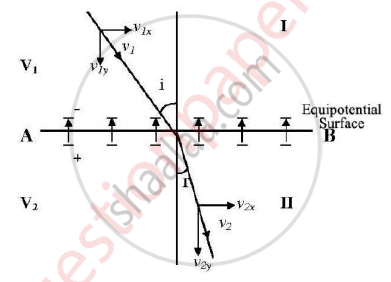Advertisement Remove all ads

# Derive Bethe’S Law for Electron Refraction. - Applied Physics 2

Answer in Brief

Derive Bethe’s Law for electron refraction.

Advertisement Remove all ads

#### Solution

Region I has potential V1 and region II has potential V2. The plane surface AB constitutes one of the equipotential surfaces. Let an electron with velocity v1 enter region I making an angle I with the normal. As the electron passes through the equipotential surface AB, it experiences a force which alters its velocity. Because the electric field exists only in the y-direction, the vertical component (y-component) of electron changes while the tangential component (x-component) remains constant.

𝑣1𝑥=𝑣2𝑥

𝑣1sin𝑖=𝑣2sin𝑟

sin i /sin r=v_2/v_1

If V1>V2, 𝑣1𝑦 increases while if V2>V1, 𝑣2𝑦 increases.In our case we have taken V2>V1. As the electrons move through the electric field their kinetic energy is provided by the respective potential energy of the electric fields. Hence mv12/2=qV1

And mv22/2=qV2

Dividing the above equation we get,

v12/ v22= V1/ V2

v1/ v2=sqrt(V1//V2)

Hence we get,sin i/sin r=(v2)/(v1)=sqrt((V2)/(V1))

This is known as Bethe’s law of electron refraction.

Concept: Electron Refraction
Is there an error in this question or solution?
Advertisement Remove all ads

#### APPEARS IN

Advertisement Remove all ads
Advertisement Remove all ads
Share
Notifications

View all notifications

Forgot password?